## Abstract

With the evolution of the electricity market into a restructured smart version, load forecasting has emerged as an eminent research domain. Many forecasting models have been proposed by researchers for electricity price and load forecasting. This state of art introduces a load time series modeled with a hybrid technique culminating from the logical amalgamation of GARCH, a conventional hard computing method, Fuzzy ARTMAP, an artificial intelligence-based soft computing technique, and wavelet transform, for treating the load time series. The study investigates into the ability of the proposed hybrid model in tackling the electricity load time series forecasting problems. The work under this study also includes comparisons drawn among models which use either one or two of the mentioned techniques and the model proposed. Results certify the efficacy and effectiveness of the model over others.

## Introduction

Forecasting [1, 2] knowingly or unknowingly holds an integral stature in every persons life. We often predict stock market, earthquake and weather in our day to day life. A business manager forecasts product sales . People make future plans based on these forecasts. Here it should be understood that it is an impossible task to make exact forecasts, we can only work incessantly towards attaining higher accuracies. Generally forecasting presumes that future occurrences depend upon past or present observable events; it assumes that some aspects of the past pattern will continue in the future. Through observing and studying past data relationships can be established between the event and the parameters persisting at the then moment .

Forecasting with load time series is a challenging application because load time series are inherently nonstationary , deterministically chaotic, and highly noisy by nature. Above this by no technique the past information can solely determine the futuristic behavior of the electricity market. Therefore, to maintain global competitiveness, market dependency on advance computer technologies is increasing day by day.

Forecasting can either be spatial or temporal in nature. While spatial forecasts are based on area covered, temporal are based on time horizon utilized. Temporal forecasting can further be divided into three subcategories: Short Term, Medium Term and Long Term . Out of these, short-term forecasting is of most importance because of its utility. They help plan capacity building, estimate load flows and prevent overloading to name a few. Forecasting techniques primarily use either statistical tools [7, 8] or artificial intelligence based algorithms .

In this study, we present a new method of forecasting electricity loads using Fuzzy ARTMAP (FA) and GARCH along with wavelet transform (WT) for day-ahead forecasting. The approach implemented aims to develop a sturdy, precise and efficient day-ahead load forecasting tool utilizing data filtering technique, employing WT, fused with a computational model implementing conjointly FA (soft computing model), and GARCH (hard computing model). Comparison results of the proposed models' performance with that utilizing only FA and utilizing both FA and GARCH show a convincing reduction in mean absolute percentage error (MAPE). A simple artificial neural network (ANN) model utilizing the same data has also been implemented simply to draw a wider range comparison and highlight the supremacy of the hybrid model over ANN.

The procedure of forecasting proposed can be summed in three steps:

1. Decomposition of past load series using WT.
2. Using FA model fitted to one approximated and two decomposed series of WT and using GARCH on one decomposed series of WT.
3. Then using inverse WT to reconstruct the forecasted load series.

The literature of the study is arranged as follows:

• Section II details WT, FA, and GARCH techniques in brief to enhance reader understanding.
• Section III elaborates on the implemented hybrid methodology.
• Section IV comprises numerical and graphical results and Section V concluding the study.

Any load data series embodies numerous spikes, nonlinearities, and fluctuations. The 2010 hourly New South Wales electricity load series is no exception to it. As illustrated in Figure 1, it is also characterized by chaotic and random changes. This series of 2148 h has been utilized in this study. Where the hourly data of 92 days or 3 months has been used to train the network and the data of next 24 h has been reserved as validation set data and is also the predicted subset.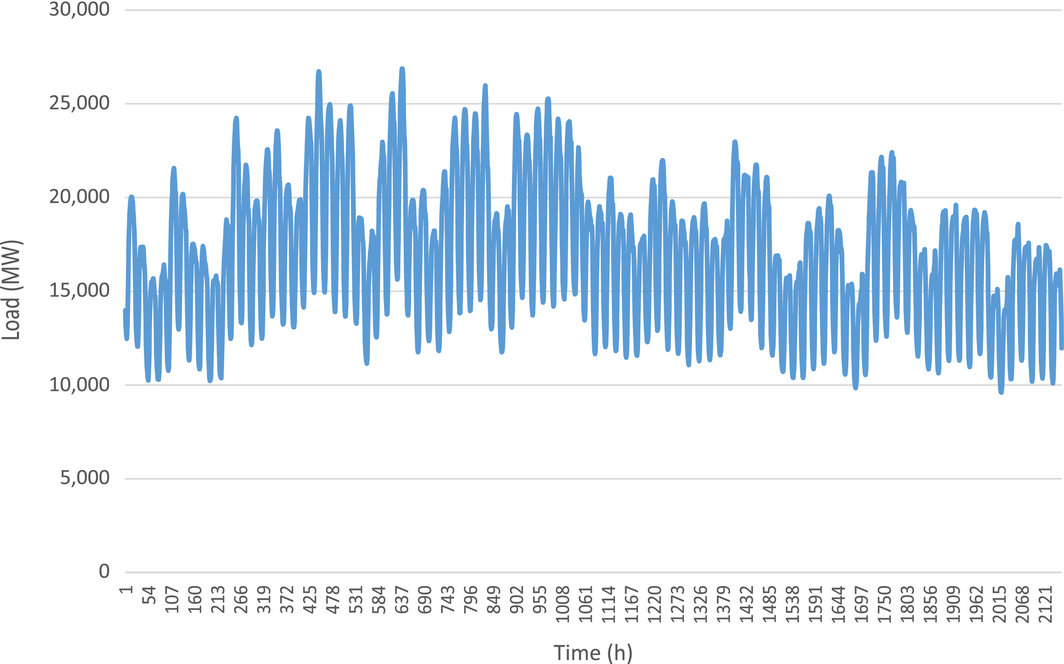Figure 1. Load data of New South Wales.

## Wavelet Transform

Wavelet transform is a mathematical model which transforms the original load series (in time domain) into constituent subseries over time domain of a different scale for processing and analysis. WT is most suitable for the nonstationary data (mean and autocorrelation of series are not constant). It is also well known that most of the load data series are nonstationary, hence the utility of WT . The WT is used to decompose the original load series into several other series with resolution of different levels, which is called multiresolution decomposition [11, 12].

Fourier transform (FT) decomposes the original load series into linear combinations as sine and cosine functions whereas by WT the series is decomposed into a sum of more flexible functions which are localized in both time and frequency .

Wavelet transform can be classified into two: continuous wavelet transform (CWT) and discrete wavelet transform (DWT).

The CWT of a continuous time signal x (t) is defined as [4, 6]:

 ${\psi }_{a,b}\left(t\right)\quad \quad =\int _{-\infty }^{\infty }x(t){\psi }_{a,b}^{_{\ast }}\left(t\right)dt,$
(1)

where ψ (t) is the mother wavelet, given by eq. (2) where a acts as a scaling parameter and b as a translating parameter.

 ${\psi }_{a,b}^{_{\ast }}\left(t\right)\quad ={\frac {1}{\sqrt {a}}}{\psi }^{_{\ast }}\left({\frac {t-b}{a}}\right).$
(2)

Each wavelet is formulated by scaling and translating the mother wavelet. The mother wavelet is an oscillatory function characterized by zero average and finite energy.

 ${\psi }_{c,d}\quad =\sum _{n}x\left(n\right){\psi }_{c,d}^{_{\ast }}\left(n\right),$
(3)

where

 ${\psi }_{c,d}^{_{\ast }}\left(n\right)\quad ={\frac {1}{\sqrt {a_{o}^{c}}}}{\psi }^{_{\ast }}\left({\frac {n-db_{o}a_{o}^{c}}{a_{o}^{c}}}\right).$
(4)

Here, c acts as a scaling coefficient while d acts as a sampling one.

To implement DWT as a filter, Mallat propounded an algorithm called Mallat multiresolution analysis or the Mallat algorithm . It is a two-staged algorithm where decomposition occurs in the first stage followed by reconstruction in the second one. This study implements a three-level decomposition on the original load series yielding three detailed series (D) and one approximated series (A) as illustrated in Figure 2. Decomposing and reconstructing processes both involve filtering for which both high–pass (HPF) and low-pass filters (LPF) are utilized. While down-sampling occurs during wavelet decomposition, up-sampling and filtering is used in wavelet reconstruction. A Daubenchies wavelet function of order 5 (db5) has been utilized in this study as a mother wavelet.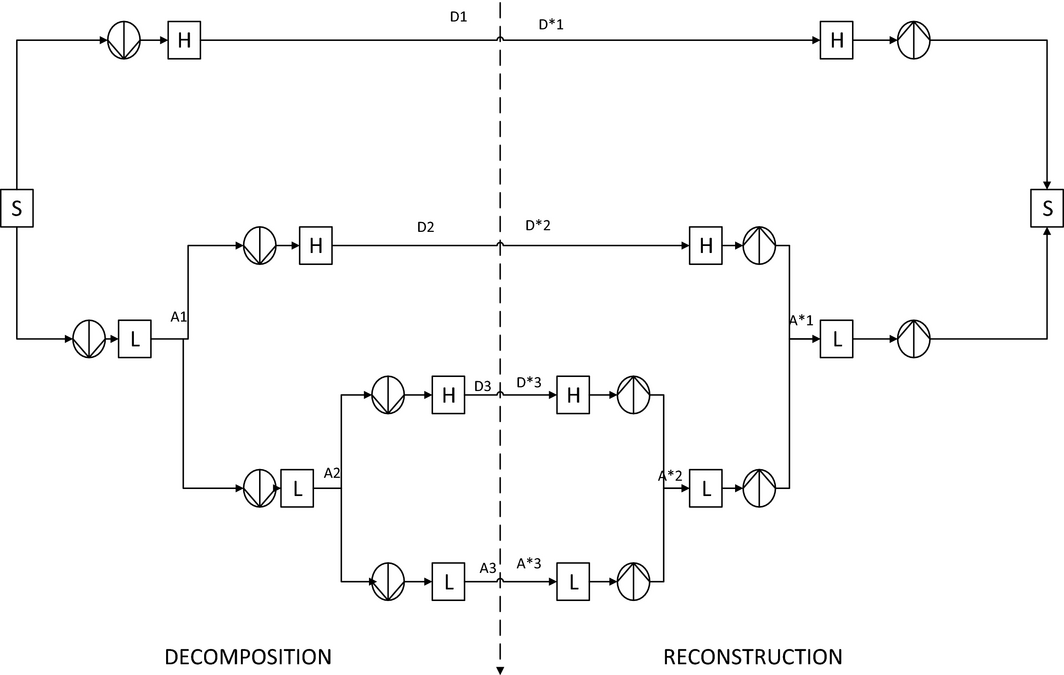Figure 2. Wavelet transformation (order 3) [23, 28].

## Fuzzy ARTMAP

The FA network is a supervised learning method based on adaptive resonance theory (ART) . FA network carries out learning without forgetting previously learned information . FA is flexible and adaptive to changes in the environment and is self-organizing by nature . FA network is a recent technique that has been utilized in forecasting applications including load forecasting.

Neural network is another popular artificial intelligence technique utilized in forecasting applications. Most neural networks struggle with the plasticity–stability dilemma which probes into ways by which a network can endure adaptive-ness or plasticity toward new inputs while staying aloof of the noisy data inputs, hence stability [17, 18]. A general neural network encounters hindrance in preserving previously learned knowledge while learning newer concepts. The FA confronts this dilemma with a feedback mechanism laid between the competitive and input layers to allow fresh concepts to be absorbed without losing the knowledge attained previously. This results in a firmer learning environment endowed with faster convergence capability compared to traditional soft computing techniques . This is also confirmed by the results of this study. These properties of FA can improve load forecasting performance as load series data are highly stochastic by nature (Figure 1).

The functional layout of FA network is shown in Figure 3. An ARTMAP system embodies twin art modules (ARTa and ARTb) to fabricate stable recognition categories corresponding to the arbitrary input patterns. ARTa uses ART-1, a type of ART network which accepts only binary input, while ARTb uses FUZZY ART. This setup enables to switch the binary module notations into a corresponding feature in the fuzzy ART module. For example, the intersection operator (^) of ART1 is replaced by the operator (^) in FUZZY ART. The architecture called FA is achieved by the synthesis of fuzzy logic and ART neural network, employing a close formal similarity between two computations of fuzzy subsets and ART category. Also, FA actualizes a new min–max learning rule that collectively minimizes predictive error and maximizes generalization, or code compression. This is achieved by a match tracking process that increases the ART vigilance parameter by the minimum amount needed to correct a predictive error. As a result, the system automatically learns a minimal number of recognition categories, or “hidden units,” to meet the criteria of accuracy. Category proliferation is prevented by normalizing input vectors at a preprocessing stage. A normalization procedure called complement coding  leads to a symmetric theory in which the AND operator (^) and the OR operator (v) of fuzzy logic plays complementary roles. In training, the best matching category is :Figure 3. Functional layout of Fuzzy ARTMAP [15, 20, 28].
 $J\quad =\quad arg{\underset {0\leq j\leq N}{max}}T_{j}\left(I_{tr}\right),$
(5)

where

 $T_{j}\left(I_{tr}\right)\quad =\quad {\begin{array}{cc}{\frac {\vert {I_{tr}}^{\wedge }W_{j}\vert }{\alpha +\vert W_{j}\vert }}&,{\mbox{If}}\quad {\frac {\vert I_{tr}{}^{\wedge }W_{j}\vert }{\vert I_{tr}\vert }}\geq \rho \\,&{\mbox{Otherwise}}\end{array}}$
(6)

where Tj = choice function, α = choice parameter, ^ = Fuzzy MIN operator, ρ = vigilance parameter, and ${\textstyle {\frac {\left|I_{tr}\wedge W_{j}\right|}{\left|I_{tr}\right|}}\geq \rho }$ is the vigilance criteria. If vigilance criteria satisfy, then resonance occurs. During training, the vigilance criteria vary from baseline vigilance which is the initial value. If vigilance criteria qualify, then category J becomes representative membership function for time series, and the weighing vector of the winning category Wj is updated as per the following equation:

 $W_{j}^{\left(new\right)}=\beta \left(I_{tr}\wedge W_{j}^{\left(old\right)}\right)+$$\left(1-\beta \right)W_{j}^{\left(old\right)}.$
(7)

Here β represents the learning rate. If vigilance criteria fail, then category J is deactivated for the present load series by equating choice function equals to zero. If ARTb does not predict the correct output for ARTa, then the vigilance parameter is increased. This is called match tracking, in which the value of the vigilance parameter is slightly increased to a new value :

 $\rho \quad ={\frac {\left|I_{tr}\wedge W_{j}\right|}{\left|I_{tr}\right|}}\quad +$$\quad \epsilon ,$
(8)

where ε denotes the learning precision.

The scheme resizes a category on predictive success by amplifying the vigilance parameter ρ by a minimal amount essential to verify the predictive error in ARTb. The parameter ρ holds an inverse relationship with the category size. A lower value leads to a broadly generalized category with higher compressed code. This parameter rates the minimum faith that ARTa should have while accepting a category during hypothesis testing which focuses ARTa on a new cluster. The failures at ARTa increase ρ to that threshold value which in turn triggers ARTa under a process called match tracking. This technique reduces generalization essential to correct a predictive error. The combination of these techniques, i.e. ARTMAP and match tracking leads to a faster learning and erudition from a rare event. The fuzzy ART reduces to ART1 for a binary input and works as self for a binary input and works as self for an analog vector. Thus the crisp logics of ART1 with their fuzzy counterparts form a potent module.

Once the training stage is completed, the FA network is used as a classifier of the input load series which is given to ARTa. ARTb is not used during classification process and the learning capability of the network is deactivated during classifying process (i.e. β = 0). In this stage we get predicted classified labels in the output of ARTMAP. These classified labels are later de-fuzzified to get the forecasted loads.

## GARCH

GARCH stands for Generalized Autoregressive Conditional Hetero-skedasticity which is used to model observed time series. GARCH is effectively implemented to highly volatile time series caused by unexpected random effects [20, 21]. The model GARCH (p, q) is defined as:

 $x_{t}=\mu +{\epsilon }_{t},$
(9)

where μ is offset and εt = σtzt.

Considering a time series xt with a constant mean offset :

 ${\sigma }_{t}^{2}\quad =\quad c\quad +\quad \sum _{i\quad =1}^{q}{\phi }_{i}{\epsilon }_{t-i}^{2}\quad +$$\quad \sum _{i\quad =1}^{p}{\psi }_{i}{\sigma }_{t-i}^{2},$
(10)

where p is the order of GARCH terms σ2 and q is the order of ARCH terms ε2.

As can be seen in eq. (10), in GARCH (p, q) model is p = 0, i.e. a GARCH (0, q) model becomes an ARCH (q) model.

A limitation of the GARCH model is that it can only be specified for stationary time series, hence the below equation must be satisfied for stationary time series only:

 $\sum _{i=1}^{q}{\phi }_{i}+\sum _{i=1}^{p}{\psi }_{i}>1.$
(11)

Steps for GARCH modeling [21, 22]:

1. Identify the class of models.
2. Identify the subsets of GARCH models.
3. Parameter estimation.
4. Model validation.
5. Apply forecast.

## Proposed Methodology

Figure 4 presents the schematic diagram of the prospective hybrid model for day-ahead electricity load forecasting built on the FA technique combined with GARCH and WT. The procedure for forecasting is as follows: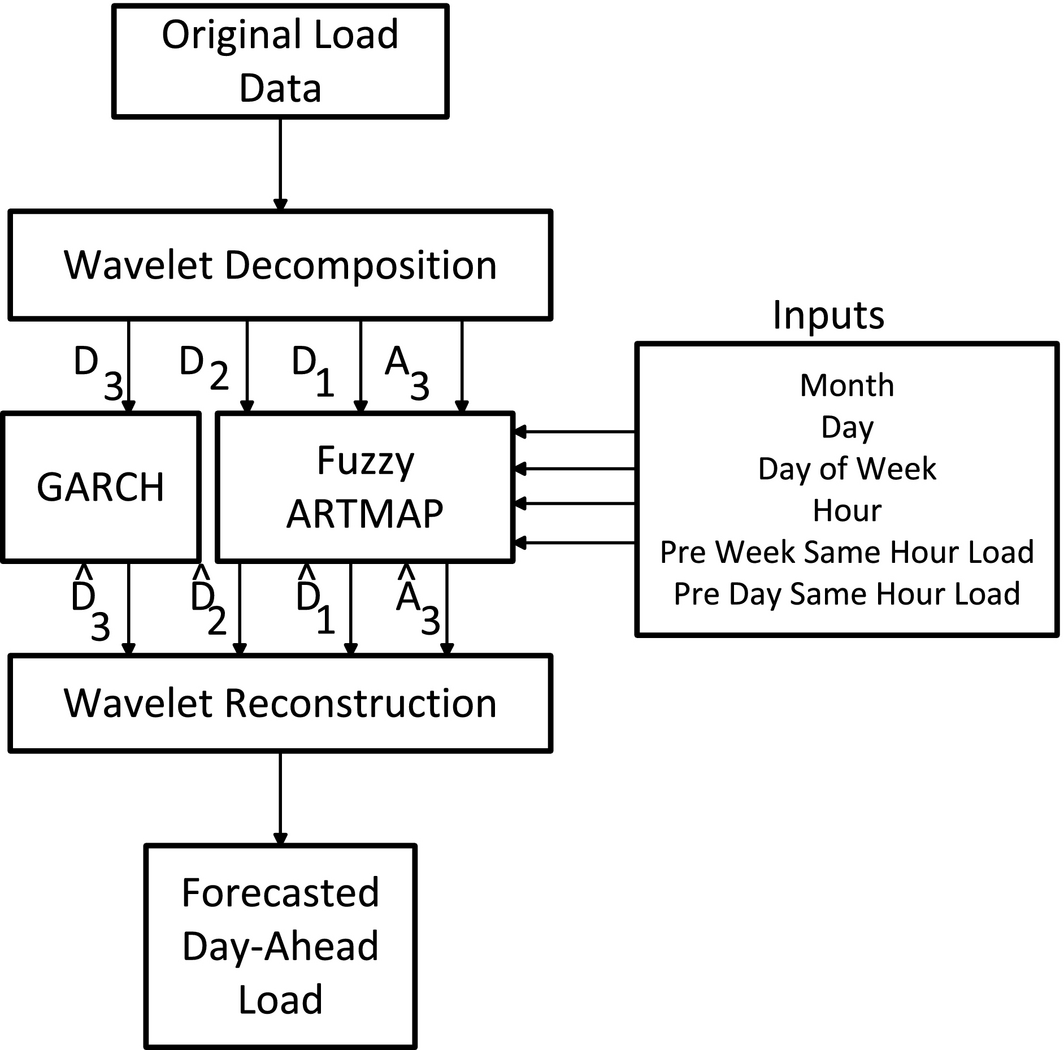Figure 4. Schematic diagram of the proposed hybrid model.
1. In the proposed hybrid model, input variables are hourly data of electricity load, month, day, day of week, hour, previous week same hour load and previous day same hour load. Only the load series is passed through WT. The load series is decomposed through WT into four components. The decomposed detailed coefficients, D1, D2, D3 (high frequency components) and approximated series, A3 (low frequency components) are then obtained by down sampling with HPF and LPF, respectively.
2. The characteristic decomposed load series (D1, D2, and A3) along with other input data are furnished into the FA network and decomposed load series D3 is fed into GARCH.
3. The output components of GARCH, FA network, and the decomposed detailed and approximate series are then processed by wavelet reconstruction to produce the day-ahead electricity loads.

This step-by-step summary is shown in Figure 5.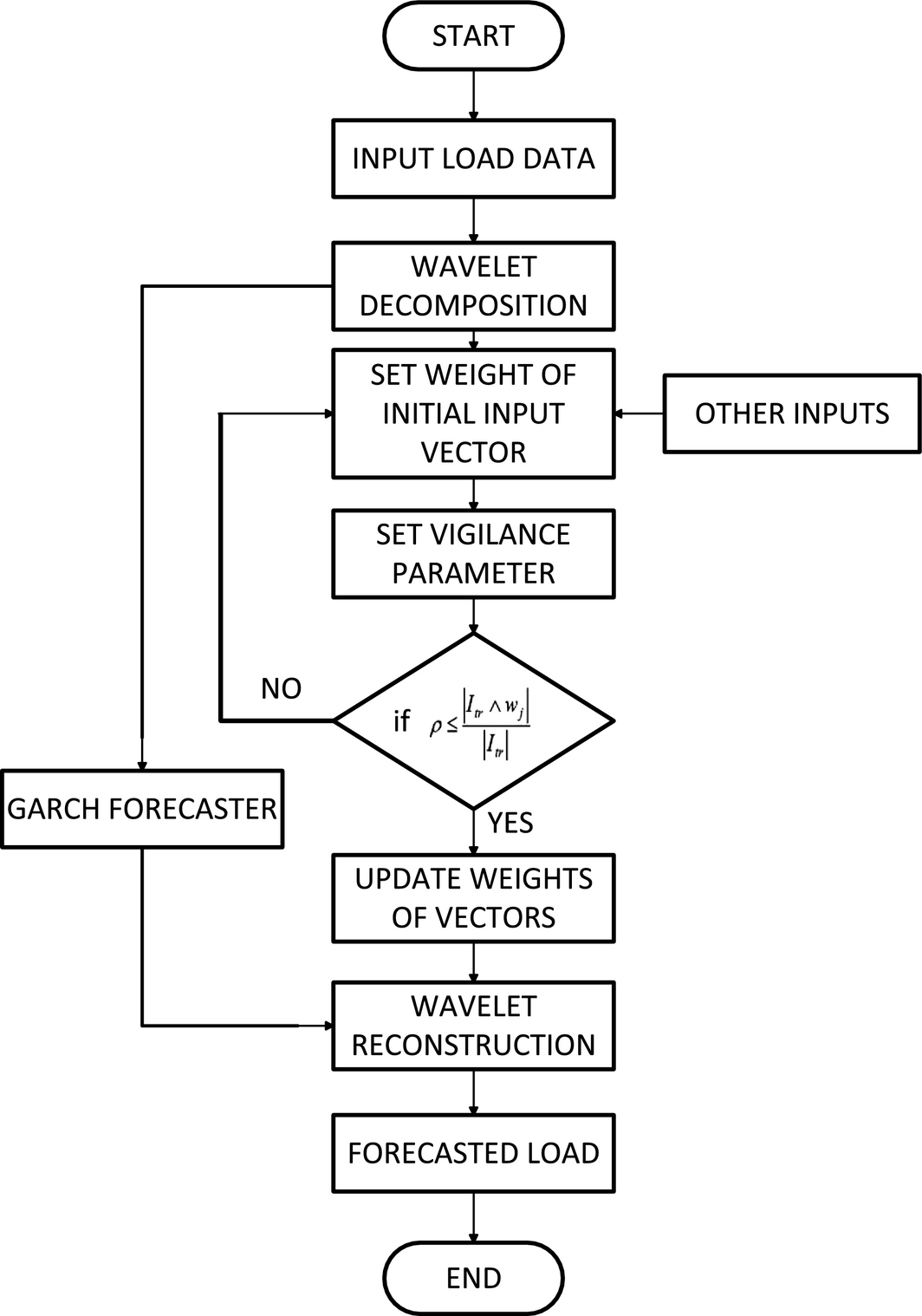Figure 5. Flowchart for the proposed method for day-ahead load forecasting.

## Numerical and Graphical Results

The paper introduces a new hybrid algorithm based on WT, FA, and GARCH, which accounts for the interactions of month, day, day of week, hour, previous week same hour load, and previous day same hour load. The proposed method has worked on the electricity load data of New South Wales. To rank the performance of the proposed model, the results have been compared to other models such as FA, FA + WT, and the most employed artificial intelligence technique, ANN. The summary table of this is illustrated in the 'Conclusion' section.

Above this the outputs of the mentioned models have been tabulated below followed by the respective graphs comparing the forecasted and actual data.

Before one takes a look at these, we have briefed how they have been tabulated and why they serve as an appropriate measure of the efficiency of any forecasting model. Error is defined as the difference between the actual value and the forecasted value for the corresponding period [10, 23-26].

 ${\epsilon }_{t}\quad =\quad A_{t}\quad -\quad F_{t},$
(12)

where εt is the error for the period t, At is the actual value for the period t, and Ft is the forecasted value for the period t. MAPE or mean average percentage error is the most widely accepted parameter of forecasting error, which mathematically means:

 ${\mbox{MAPE}}\quad =\quad {\frac {\sum _{t=1}^{N}\left|{\frac {{\epsilon }_{t}}{A_{t}}}\right|}{N}}.$
(13)

In this study, N has been valued 24 for daily electricity load forecasts. N should be valued 168 when we attempt weekly electricity forecasts. The graph shown below uses N = 24 as it predicts the day-ahead forecasts. Figures 6-9 represent the actual versus forecasted data for FA, FA + WT, FA + WT + GARCH, and ANN, respectively. Table 1 presents the actual versus forecasted data for all the techniques, namely FA, FA + Wavelet, ANN, and the proposed model (FA + WT + GARCH).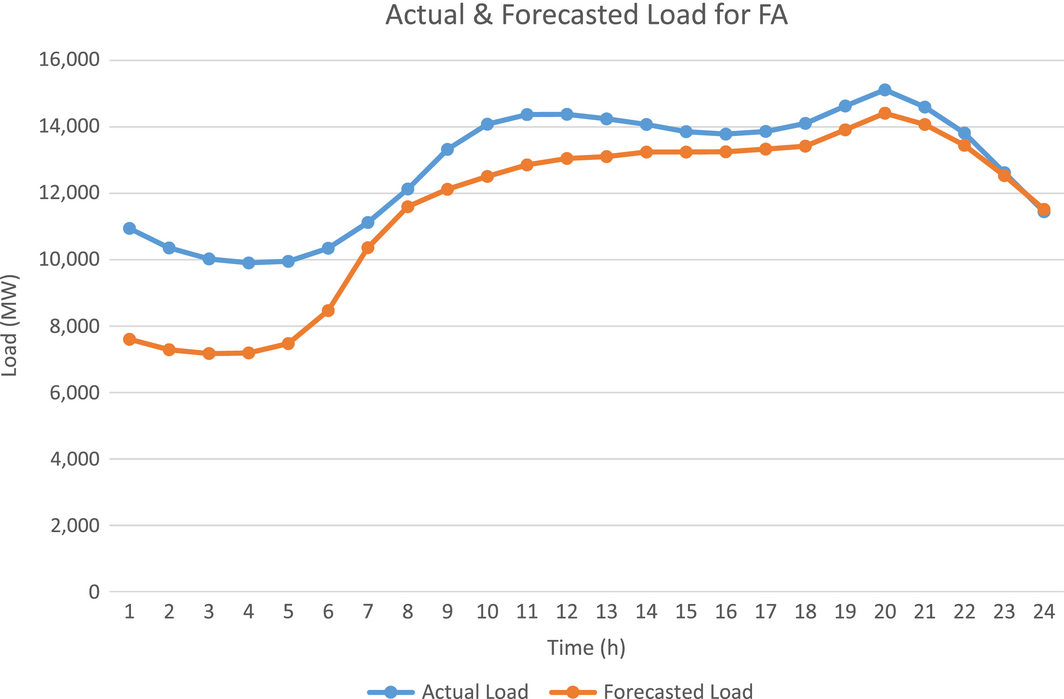Figure 6. Actual and forecasted load for FA. FA, Fuzzy ARTMAP.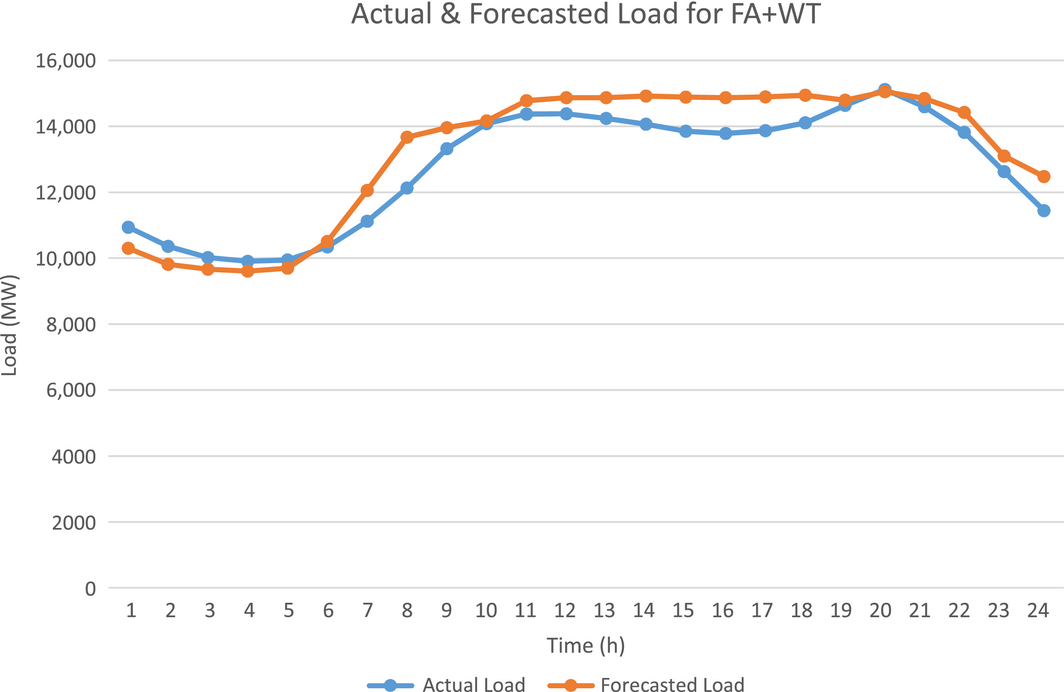Figure 7. Actual and forecasted load for FA + WT. FA, Fuzzy ARTMAP; WT, wavelet transform.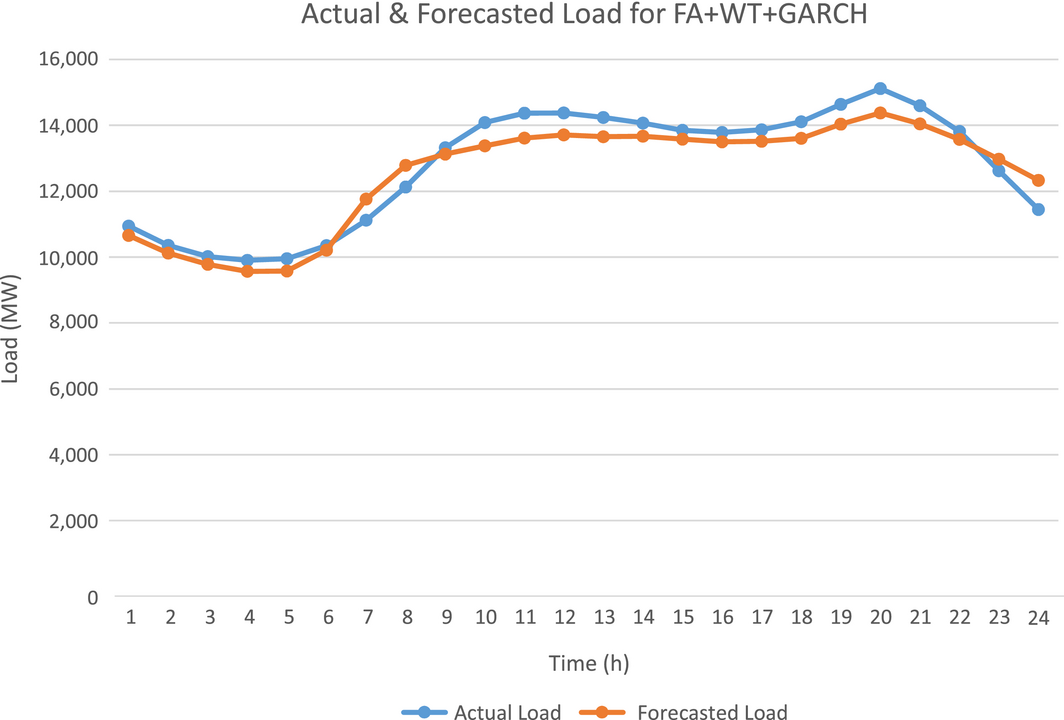Figure 8. Actual and forecasted load for FA + WT + GARCH. FA, Fuzzy ARTMAP; WT, wavelet transform.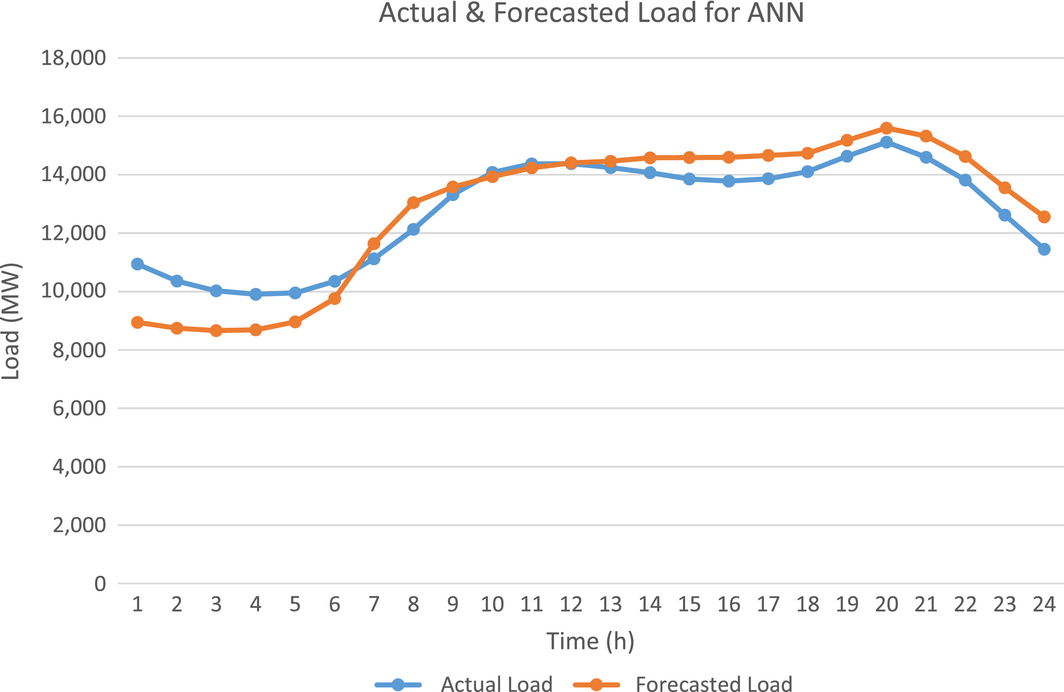Figure 9. Actual and forecasted load for artificial neural network.

Table 1. Comparative hourly forecasts for respective techniques
Hours Actual load FA FA + WT FA + WT + GARCH ANN
1. ANN, artificial neural network; FA, Fuzzy ARTMAP; MAPE, mean absolute percentage error; WT, wavelet transform.

1 10940 7599.86 30.53 10304.45 5.81 10654.79 2.61 8945.86 18.23
2 10357 7285.57 29.66 9810.04 5.28 10124.10 2.25 8740.28 15.61
3 10020 7171.58 28.43 9668.12 3.51 9777.74 2.42 8660.86 13.56
4 9906 7190.78 27.41 9607.85 3.01 9566.39 3.43 8691.42 12.26
5 9950 7476.25 24.86 9700.96 2.50 9575.59 3.76 8961.52 9.93
6 10347 8463.64 18.20 10508.24 −1.56 10209.69 1.33 9762.46 5.65
7 11119 10358.68 6.84 12054.61 −8.41 11765.89 −5.82 11636.54 −4.65
8 12130 11593.56 4.42 13668.34 −12.68 12787.44 −5.42 13048.84 −7.57
9 13322 12118.27 9.04 13958.09 −4.77 13122.40 1.50 13583.11 −1.96
10 14081 12509.26 11.16 14160.53 −0.56 13374.91 5.01 13937.94 1.02
11 14369 12851.78 10.56 14778.85 −2.85 13611.55 5.27 14231.49 0.96
12 14376 13049.30 9.23 14868.05 −3.42 13708.11 4.65 14402.53 −0.18
13 14241 13104.27 7.98 14868.00 −4.40 13651.71 4.14 14458.80 −1.53
14 14069 13240.01 5.89 14914.21 −6.01 13668.93 2.84 14580.21 −3.63
15 13852 13243.24 4.39 14887.22 −7.47 13578.73 1.97 14589.42 −5.32
16 13783 13252.21 3.85 14864.27 −7.84 13503.08 2.03 14596.21 −5.90
17 13864 13331.54 3.84 14892.18 −7.42 13519.43 2.49 14663.60 −5.77
18 14107 13415.88 4.90 14943.05 −5.93 13601.57 3.58 14734.08 −4.45
19 14633 13908.10 4.95 14796.38 −1.12 14039.43 4.06 15180.97 −3.74
20 15117 14411.75 4.67 15048.74 0.45 14379.41 4.88 15598.05 −3.18
21 14595 14069.20 3.60 14845.71 −1.72 14042.48 3.79 15325.88 −5.01
22 13813 13441.37 2.69 14420.63 −4.40 13572.74 1.74 14628.50 −5.90
23 12623 12529.19 0.74 13097.21 −3.76 12974.20 −2.78 13556.04 −7.39
24 11446 11518.88 −0.64 12474.47 −8.99 12333.07 −7.75 12557.34 −9.71
Overall MAPE     10.77   4.74 3.56     6.38

## Conclusion

The hybrid model proposed in this paper is for short-term electricity load forecasting. The model is the aftermath of befitting coalition of FA, GARCH, and WT. While WT looks after the ill-behaved load series, FA captures the nonlinear fluctuations by virtue of stability–plasticity dilemma . The attributes of FA renders the proposed hybrid method robustness and higher efficiency enabling forecasting meeting higher accuracy.

The model has also been compared with FA, FA + WT, and ANN. The results certify the efficacy of the proposed load forecasting hybrid model, as can be seen from Table 2.

Table 2. Mean absolute percentage error comparison of various models for load
MAPE of various models
ANN FA FA + WT FA + WT + GARCH
1. ANN, artificial neural network; FA, Fuzzy ARTMAP; MAPE, mean absolute percentage error; WT, wavelet transform.

6.381% 10.772% 4.745% 3.563%

## Acknowledgments

The authors thank the New South Wales System Operator for providing hourly data of electricity load (http://www.asx.com.au/).

## Conflict of Interest

None declared.

### Document informationPublished on 01/06/17
Submitted on 01/06/17

Licence: Other

### Document Score0

Views 41
Recommendations 0